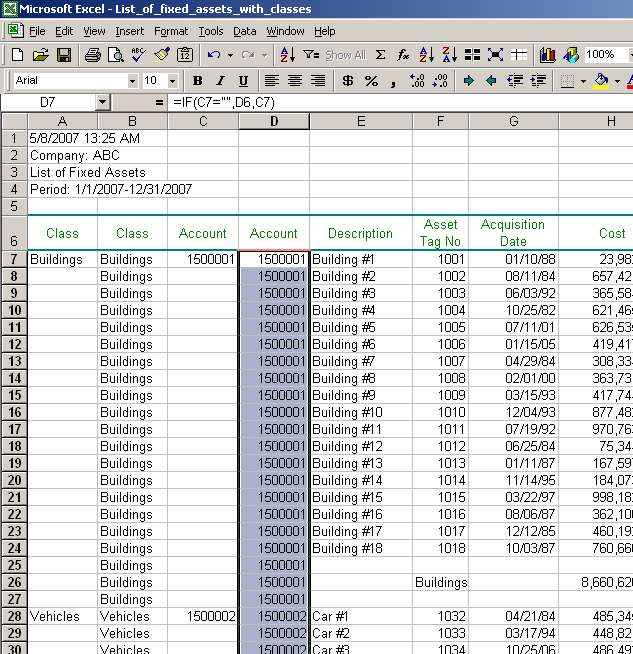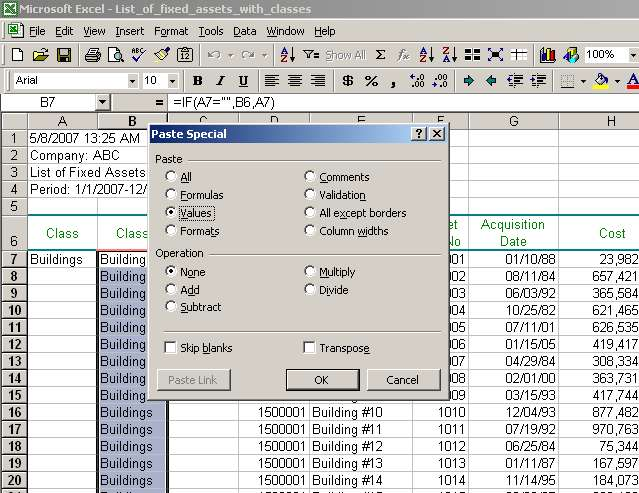# Excel Fill Down Function, Excel Fill Down Examples

We can see that when we copied the formula, values in cells B7-B27 equal "Building." Then, in row 28, the value changes to "Vehicle"; in row 55, the value changes to "Equipment" and so forth. So, what's happening? The formula assigns a value in column B from a cell right above it, unless there is a non-empty cell in column A. For example, cell B27 has a value equal to "Building" which is taken from cell B26. Value in cell B26 is taken from cell B25 and so forth until cell B7. Value in cell B7 is taken from cell A7 as cell A7 is not empty.

Now, moving back down to cell B28. When the formula is pasted to cell B28, it determines that cell A28 is not empty and thus, assigns value of cell A28 to cell B28 instead of assigning value from cell B27. Next, formula in cell B29 checks that value in cell A29 is an empty string and thus, assigns value of cell B28 to cell B29. Then the process continues (copying value of a cell above), until the formula hits row 55 where the value switches to "Equipment" and so on.

The result is exactly what we wanted - empty cells filled in with their respective values. We can perform the same procedure for column Account. To do that, let us copy cell B7 to cells D7-D191. We get the same result as in column Class, but now for column Account. Note that because we had relative references in cell B7 (meaning, references to cells shift when we copy a formula in another cell), we did not have to make any adjustments to the formula:### 5) Clean up the file

Once we are done filling in the empty cells, we should clean up the file and delete unnecessary / rudimentary information from it. First, let us copy and paste values in columns B and D. As of now, values in those two columns are dependent on values in columns A and C. If anything changes in columns A and C (see below where we delete those columns), values in columns B and D will change as well. We don't want that to happen. To prevent that, we paste values (values are not dependent on any cell) in columns B and D.

To copy and paste values, select cells B7-B191 and copy them by using key combination Ctrl+C. Now, we need to paste values. Go to menu Edit, select Paste Special. In the dialog box, select Values (see tab Step 5-1 in our listing of fixed assets):Click OK. You will see that values in cells B7-B191 changed from formulas to text strings.

Complete the same procedure for values in column D.

Page 5 of 6